# nLab 4T relation

Contents

### Context

#### Knot theory

knot theory

Examples/classes:

Types

knot invariants

Related concepts:

category: knot theory

# Contents

## Idea

### For round chord diagrams

In knot theory by the 4-term relations or 4T-relations, for short, one means the following relations in the linear span of chord diagrams: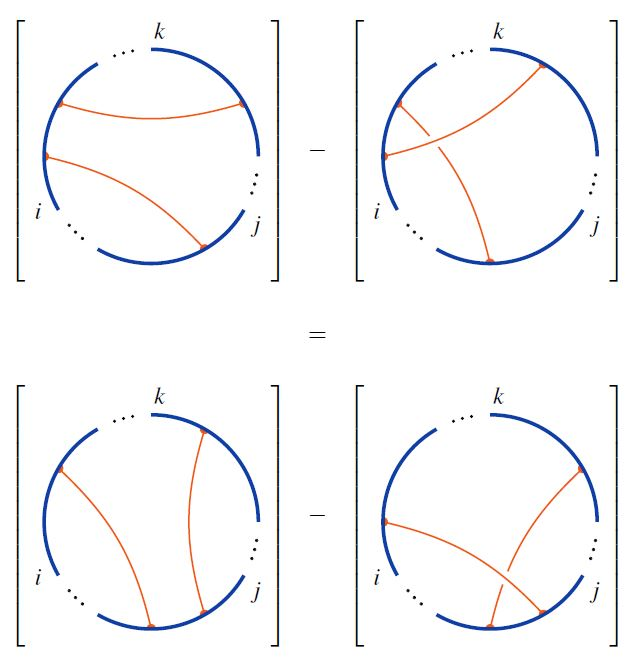graphics from Sati-Schreiber 19c

These are the relations respected by weight systems on chord diagrams.

### For horizontal chord diagrams

For horizontal chord diagrams the 4T relations is the following: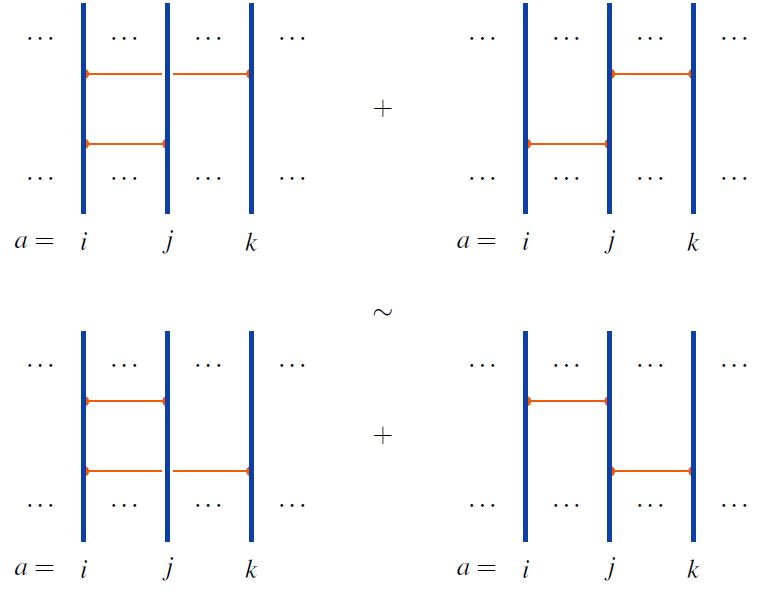graphics from Sati-Schreiber 19c

When the linear span of horizontal chord diagrams is regarded as an associative algebra under concatenation of strands (here), this relation is the infinitesimal braid relation

$\big[ t_{i k} + t_{j k} \,,\, t_{i j} \big] \;=\; 0 \,.$

## Properties

### Relation between horizontal and round 4T relations

The 4T-relations for round chord diagrams are the image of the 4T relations for horizontal chord diagrams under tracing horizontal to round chord diagrams: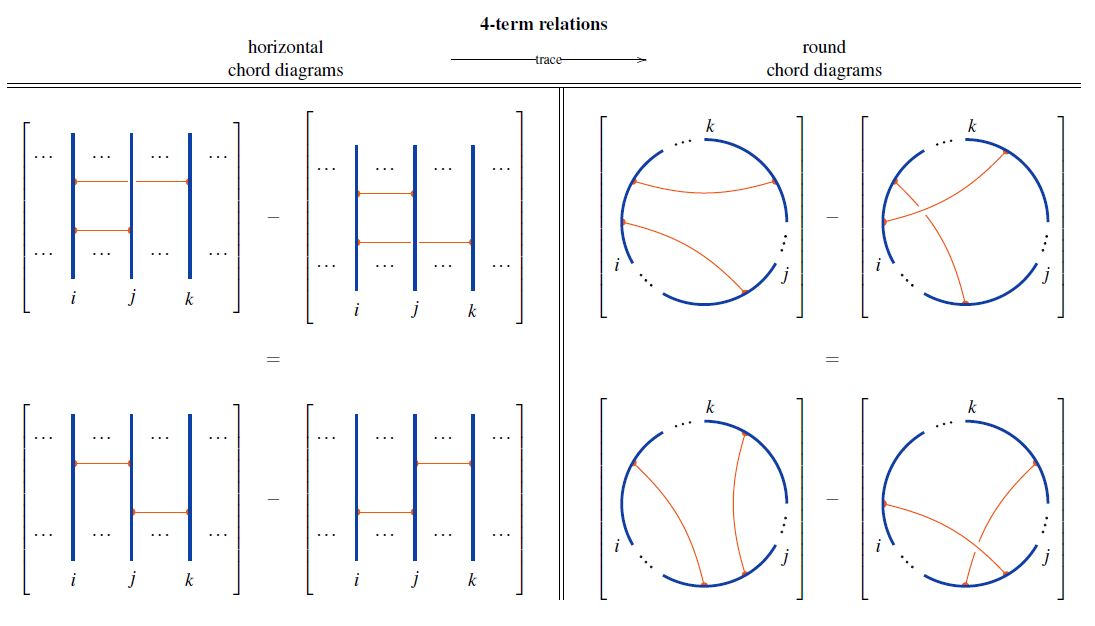### Relation to STU-relations

Under the embedding of the set of round chord diagrams into the set of Jacobi diagrams, the STU-relations imply the 4T relations on round chord diagrams: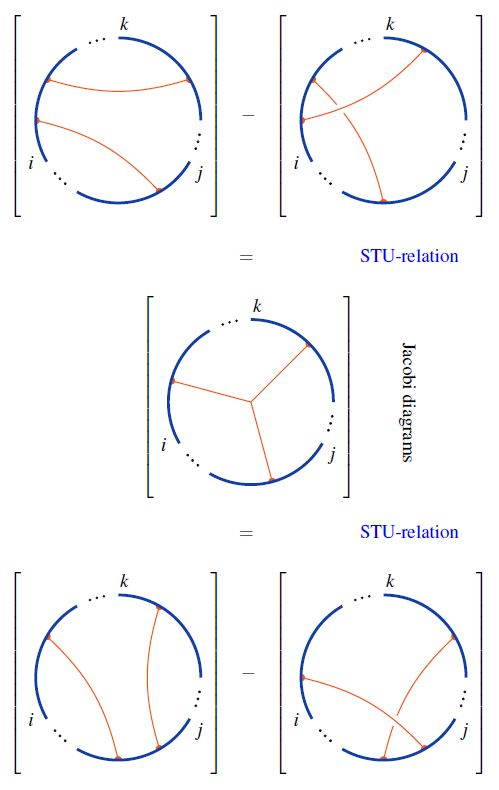Using this, one finds that chord diagrams modulo 4T are Jacobi diagrams modulo STU: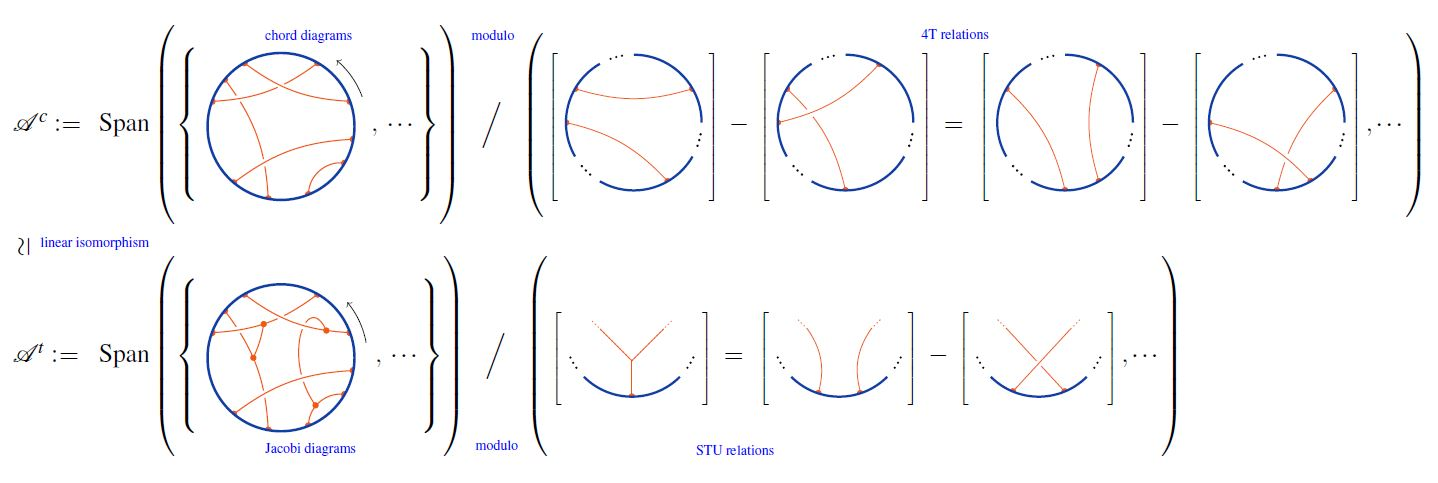graphics from Sati-Schreiber 19c

Original articles

Textbook accounts

Lecture notes:

The graphics above are taken from Sati-Schreiber 19.